Theory and Modern Applications

# Stability of general multi-Euler-Lagrange quadratic functional equations in non-Archimedean fuzzy normed spaces

## Abstract

In this paper we prove the generalized Hyers-Ulam stability of the system defining general Euler-Lagrange quadratic mappings in non-Archimedean fuzzy normed spaces over a field with valuation using the direct and the fixed point methods.

MSC:39B82, 39B52, 46H25.

## 1 Introduction

Let $\mathbb{K}$ be a field. A valuation mapping on $\mathbb{K}$ is a function $|\cdot |:\mathbb{K}\to \mathbb{R}$ such that for any $r,s\in \mathbb{K}$ the following conditions are satisfied: (i) $|r|\ge 0$ and equality holds if and only if $r=0$; (ii) $|rs|=|r|\cdot |s|$; (iii) $|r+s|\le |r|+|s|$.

A field endowed with a valuation mapping will be called a valued field. The usual absolute values of $\mathbb{R}$ and $\mathbb{C}$ are examples of valuations. A trivial example of a non-Archimedean valuation is the function $|\cdot |$ taking everything except for 0 into 1 and $|0|=0$. In the following we will assume that $|\cdot |$ is non-trivial, i.e., there is an ${r}_{0}\in \mathbb{K}$ such that $|{r}_{0}|\ne 0,1$.

If the condition (iii) in the definition of a valuation mapping is replaced with a strong triangle inequality (ultrametric): $|r+s|\le max\left\{|r|,|s|\right\}$, then the valuation $|\cdot |$ is said to be non-Archimedean. In any non-Archimedean field we have $|1|=|-1|=1$ and $|n|\le 1$ for all $n\in \mathbb{N}$.

Throughout this paper, we assume that $\mathbb{K}$ is a valued field, $\mathcal{X}$ and $\mathcal{Y}$ are vector spaces over $\mathbb{K}$, $a,b\in \mathbb{K}$ are fixed with $\lambda :={a}^{2}+{b}^{2}\ne 0,1$ (${\lambda }_{1}:=2a\ne 0,1$ if $a=b$) and n is a positive integer. Moreover, $\mathbb{N}$ stands for the set of all positive integers and $\mathbb{R}$ (respectively, $\mathbb{Q}$) denotes the set of all reals (respectively, rationals).

A mapping $f:{\mathcal{X}}^{n}\to \mathcal{Y}$ is called a general multi-Euler-Lagrange quadratic mapping if it satisfies the general Euler-Lagrange quadratic equations in each of their n arguments: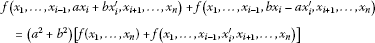(1.1)

for all $i=1,\dots ,n$ and all ${x}_{1},\dots ,{x}_{i-1},{x}_{i},{x}_{i}^{\prime },{x}_{i+1},\dots ,{x}_{n}\in \mathcal{X}$. Letting ${x}_{i}={x}_{i}^{\prime }=0$ in (1.1), we get $f\left({x}_{1},\dots ,{x}_{i-1},0,{x}_{i+1},\dots ,{x}_{n}\right)=0$. Putting ${x}_{i}^{\prime }=0$ in (1.1), we have

$f\left({x}_{1},\dots ,{x}_{i-1},a{x}_{i},{x}_{i+1},\dots ,{x}_{n}\right)+f\left({x}_{1},\dots ,{x}_{i-1},b{x}_{i},{x}_{i+1},\dots ,{x}_{n}\right)=\lambda f\left({x}_{1},\dots ,{x}_{n}\right).$
(1.2)

Replacing ${x}_{i}$ by $a{x}_{i}$ and ${x}_{i}^{\prime }$ by $b{x}_{i}$ in (1.1), respectively, we obtain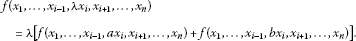(1.3)

From (1.2) and (1.3), one gets

$f\left({x}_{1},\dots ,{x}_{i-1},\lambda {x}_{i},{x}_{i+1},\dots ,{x}_{n}\right)={\lambda }^{2}f\left({x}_{1},\dots ,{x}_{n}\right)$
(1.4)

for all $i=1,\dots ,n$ and all ${x}_{1},\dots ,{x}_{n}\in \mathcal{X}$. If $a=b$ in (1.1), then we have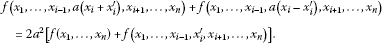(1.5)

Letting ${x}_{i}^{\prime }={x}_{i}$ in (1.5), we obtain

$f\left({x}_{1},\dots ,{x}_{i-1},{\lambda }_{1}{x}_{i},{x}_{i+1},\dots ,{x}_{n}\right)={\lambda }_{1}^{2}f\left({x}_{1},\dots ,{x}_{n}\right)$
(1.6)

for all $i=1,\dots ,n$ and all ${x}_{1},\dots ,{x}_{n}\in \mathcal{X}$.

The study of stability problems for functional equations is related to a question of Ulam  concerning the stability of group homomorphisms and affirmatively answered for Banach spaces by Hyers . The result of Hyers was generalized by Aoki  for approximate additive mappings and by Rassias  for approximate linear mappings by allowing the Cauchy difference operator $CDf\left(x,y\right)=f\left(x+y\right)-\left[f\left(x\right)+f\left(y\right)\right]$ to be controlled by $ϵ\left({\parallel x\parallel }^{p}+{\parallel y\parallel }^{p}\right)$. In 1994, a further generalization was obtained by Găvruţa , who replaced $ϵ\left({\parallel x\parallel }^{p}+{\parallel y\parallel }^{p}\right)$ by a general control function $\phi \left(x,y\right)$. We refer the reader to see, for instance, [1, 47, 1416, 18, 20, 22, 23, 25, 26, 28, 3137] for more information on different aspects of stability of functional equations. On the other hand, for some outcomes on the stability of multi-quadratic and Euler-Lagrange-type quadratic mappings we refer the reader to [7, 11, 24].

The main purpose of this paper is to prove the generalized Hyers-Ulam stability of multi-Euler-Lagrange quadratic functional equation (1.1) in complete non-Archimedean fuzzy normed spaces over a field with valuation using the direct and the fixed point methods.

## 2 Preliminaries

We recall the notion of non-Archimedean fuzzy normed spaces over a field with valuation and some preliminary results (see for instance [3, 22, 23, 31, 32]). For more details the reader is referred to [3, 22].

Definition 2.1 Let $\mathcal{X}$ be a linear space over a field $\mathbb{K}$ with a non-Archimedean valuation $|\cdot |$. A function $\parallel \cdot \parallel :\mathcal{X}\to \left[0,\mathrm{\infty }\right)$ is said to be a non-Archimedean norm if it satisfies the following conditions:

1. (i)

$\parallel x\parallel =0$ if and only if $x=0$;

2. (ii)

$\parallel rx\parallel =|r|\parallel x\parallel$, $r\in \mathbb{K}$, $x\in \mathcal{X}$;

3. (iii)

the strong triangle inequality

$\parallel x+y\parallel \le max\left\{\parallel x\parallel ,\parallel y\parallel \right\},\phantom{\rule{1em}{0ex}}x,y\in \mathcal{X}.$

Then $\left(\mathcal{X},\parallel \cdot \parallel \right)$ is called a non-Archimedean normed space. By a complete non-Archimedean normed space, we mean one in which every Cauchy sequence is convergent.

In 1897, Hensel discovered the p-adic numbers as a number-theoretical analogue of power series in complex analysis. Let p be a prime number. For any nonzero rational number a, there exists a unique integer r such that $a={p}^{r}m/n$, where m and n are integers not divisible by p. Then ${|a|}_{p}:={p}^{-r}$ defines a non-Archimedean norm on $\mathbb{Q}$. The completion of $\mathbb{Q}$ with respect to the metric $d\left(a,b\right)={|a-b|}_{p}$ is denoted by ${\mathbb{Q}}_{p}$ which is called the p-adic number field. Note that if $p>2$, then ${|{2}^{n}|}_{p}=1$ for each integer n but ${|2|}_{2}<1$.

During the last three decades, p-adic numbers have gained the interest of physicists for their research, in particular, into problems deriving from quantum physics, p-adic strings, and superstrings (see for instance ).

A triangular norm (shorter t-norm, ) is a binary operation $T:\left[0,1\right]×\left[0,1\right]\to \left[0,1\right]$ which satisfies the following conditions: (a) T is commutative and associative; (b) $T\left(a,1\right)=a$ for all $a\in \left[0,1\right]$; (c) $T\left(a,b\right)\le T\left(c,d\right)$ whenever $a\le c$ and $b\le d$ for all $a,b,c,d\in \left[0,1\right]$. Basic examples of continuous t-norms are the Łukasiewicz t-norm ${T}_{L}$, ${T}_{L}\left(a,b\right)=max\left\{a+b-1,0\right\}$, the product t-norm ${T}_{P}$, ${T}_{P}\left(a,b\right)=ab$ and the strongest triangular norm ${T}_{M}$, ${T}_{M}\left(a,b\right)=min\left\{a,b\right\}$. A t-norm is called continuous if it is continuous with respect to the product topology on the set $\left[0,1\right]×\left[0,1\right]$.

A t-norm T can be extended (by associativity) in a unique way to an m-array operation taking for $\left({x}_{1},\dots ,{x}_{m}\right)\in {\left[0,1\right]}^{m}$, the value $T\left({x}_{1},\dots ,{x}_{m}\right)$ defined recurrently by ${T}_{i=1}^{0}{x}_{i}=1$ and ${T}_{i=1}^{m}{x}_{i}=T\left({T}_{i=1}^{m-1}{x}_{i},{x}_{m}\right)$ for $m\in \mathbb{N}$. T can also be extended to a countable operation, taking for any sequence ${\left\{{x}_{i}\right\}}_{i\in \mathbb{N}}$ in $\left[0,1\right]$, the value ${T}_{i=1}^{\mathrm{\infty }}{x}_{i}$ is defined as ${lim}_{m\to \mathrm{\infty }}{T}_{i=1}^{m}{x}_{i}$. The limit exists since the sequence ${\left\{{T}_{i=1}^{m}{x}_{i}\right\}}_{m\in \mathbb{N}}$ is non-increasing and bounded from below. ${T}_{i=m}^{\mathrm{\infty }}{x}_{i}$ is defined as ${T}_{i=1}^{\mathrm{\infty }}{x}_{m+i}$.

Definition 2.2 A t-norm T is said to be of Hadžić-type (H-type, we denote by $T\in \mathcal{H}$) if a family of functions $\left\{{T}_{i=1}^{m}\left(t\right)\right\}$ for all $m\in \mathbb{N}$ is equicontinuous at $t=1$, that is, for all $\epsilon \in \left(0,1\right)$ there exists $\delta \in \left(0,1\right)$ such that

The t-norm ${T}_{M}$ is a t-norm of Hadžić-type. Other important triangular norms we refer the reader to .

Proposition 2.3 (see )

1. (1)

If $T={T}_{P}$ or $T={T}_{L}$, then

$\underset{m\to \mathrm{\infty }}{lim}{T}_{i=1}^{\mathrm{\infty }}{x}_{m+i}=1\phantom{\rule{1em}{0ex}}⟺\phantom{\rule{1em}{0ex}}\sum _{i=1}^{\mathrm{\infty }}\left(1-{x}_{i}\right)<\mathrm{\infty }.$
2. (2)

If T is of Hadžić-type, then

$\underset{m\to \mathrm{\infty }}{lim}{T}_{i=m}^{\mathrm{\infty }}{x}_{i}=\underset{m\to \mathrm{\infty }}{lim}{T}_{i=1}^{\mathrm{\infty }}{x}_{m+i}=1$

for every sequence ${\left\{{x}_{i}\right\}}_{i\in \mathbb{N}}$ in $\left[0,1\right]$ such that ${lim}_{i\to \mathrm{\infty }}{x}_{i}=1$.

Definition 2.4 (see )

Let $\mathcal{X}$ be a linear space over a valued field $\mathbb{K}$ and T be a continuous t-norm. A function $N:\mathcal{X}×\mathbb{R}\to \left[0,1\right]$ is said to be a non-Archimedean fuzzy Menger norm on $\mathcal{X}$ if for all $x,y\in \mathcal{X}$ and all $s,t\in \mathbb{R}$:

(N1) $N\left(x,t\right)=0$ for all $t\le 0$;

(N2) $x=0$ if and only if $N\left(x,t\right)=1$, $t>0$;

(N3) $N\left(cx,t\right)=N\left(x,t/|c|\right)$ if $c\ne 0$;

(N4) $N\left(x+y,max\left\{s,t\right\}\right)\ge T\left(N\left(x,s\right),N\left(y,t\right)\right)$, $s,t>0$;

(N5) ${lim}_{t\to \mathrm{\infty }}N\left(x,t\right)=1$.

If N is a non-Archimedean fuzzy Menger norm on $\mathcal{X}$, then the triple $\left(\mathcal{X},N,T\right)$ is called a non-Archimedean fuzzy normed space. It should be noticed that from the condition (N4) it follows that

$N\left(x,t\right)\ge T\left(N\left(0,t\right),N\left(x,s\right)\right)=N\left(x,s\right)$

for every $t>s>0$ and $x,y\in \mathcal{X}$, that is, $N\left(x,\cdot \right)$ is non-decreasing for every x. This implies $N\left(x,s+t\right)\ge N\left(x,max\left\{s,t\right\}\right)$. If (N4) holds, then so does

(N6) $N\left(x+y,s+t\right)\ge T\left(N\left(x,s\right),N\left(y,t\right)\right)$.

We repeatedly use the fact $N\left(-x,t\right)=N\left(x,t\right)$, $x\in \mathcal{X}$, $t>0$, which is deduced from (N3). We also note that Definition 2.4 is more general than the definition of a non-Archimedean Menger norm in [23, 31], where only fields with a non-Archimedean valuation have been considered.

Definition 2.5 Let $\left(\mathcal{X},N,T\right)$ be a non-Archimedean fuzzy normed space. Let ${\left\{{x}_{m}\right\}}_{m\in \mathbb{N}}$ be a sequence in $\mathcal{X}$. Then ${\left\{{x}_{m}\right\}}_{m\in \mathbb{N}}$ is said to be convergent if there exists $x\in \mathcal{X}$ such that ${lim}_{m\to \mathrm{\infty }}N\left({x}_{m}-x,t\right)=1$ for all $t>0$. In that case, x is called the limit of the sequence ${\left\{{x}_{m}\right\}}_{m\in \mathbb{N}}$ and we denote it by ${lim}_{m\to \mathrm{\infty }}{x}_{m}=x$. The sequence ${\left\{{x}_{m}\right\}}_{m\in \mathbb{N}}$ in $\mathcal{X}$ is said to be a Cauchy sequence if ${lim}_{m\to \mathrm{\infty }}N\left({x}_{m+p}-{x}_{m},t\right)=1$ for all $t>0$ and $p=1,2,\dots$ . If every Cauchy sequence in $\mathcal{X}$ is convergent, then the space is called a complete non-Archimedean fuzzy normed space.

Example 2.6 Let $\left(\mathcal{X},\parallel \cdot \parallel \right)$ be a real (or non-Archimedean) normed space. For each $k>0$, consider

${N}_{k}\left(x,t\right)=\left\{\begin{array}{cc}\frac{t}{t+k\parallel x\parallel },\hfill & t>0,\hfill \\ 0,\hfill & t\le 0.\hfill \end{array}$

Then $\left(\mathcal{X},{N}_{k},{T}_{M}\right)$ is a non-Archimedean fuzzy normed space.

Example 2.7 (see )

Let $\left(\mathcal{X},\parallel \cdot \parallel \right)$ be a real normed space. Then the triple $\left(\mathcal{X},N,{T}_{P}\right)$, where

$N\left(x,t\right)=\left\{\begin{array}{cc}{e}^{-\parallel x\parallel /t},\hfill & t>0,\hfill \\ 0,\hfill & t\le 0\hfill \end{array}$

is a non-Archimedean fuzzy normed space. Moreover, if $\left(\mathcal{X},\parallel \cdot \parallel \right)$ is complete, then $\left(\mathcal{X},N,{T}_{P}\right)$ is complete and therefore it is a complete non-Archimedean fuzzy normed space over an Archimedean valued field.

Let Ω be a set. A function $d:\mathrm{\Omega }×\mathrm{\Omega }\to \left[0,\mathrm{\infty }\right]$ is called a generalized metric on Ω if d satisfies

1. (1)

$d\left(x,y\right)=0$ if and only if $x=y$; (2) $d\left(x,y\right)=d\left(y,x\right)$, $x,y\in \mathrm{\Omega }$; (3) $d\left(x,y\right)\le d\left(x,z\right)+d\left(y,z\right)$, $x,y,z\in \mathrm{\Omega }$.

For explicitly later use, we recall the following result by Diaz and Margolis .

Theorem 2.8 Let $\left(\mathrm{\Omega },d\right)$ be a complete generalized metric space and $J:\mathrm{\Omega }\to \mathrm{\Omega }$ be a strictly contractive mapping with Lipschitz constant $0, that is

$d\left(Jx,Jy\right)\le Ld\left(x,y\right),\phantom{\rule{1em}{0ex}}x,y\in \mathrm{\Omega }.$

If there exists a nonnegative integer ${m}_{0}$ such that $d\left({J}^{{m}_{0}}x,{J}^{{m}_{0}+1}x\right)<\mathrm{\infty }$ for an $x\in \mathrm{\Omega }$, then

1. (1)

the sequence ${\left\{{J}^{m}x\right\}}_{m\in \mathbb{N}}$ converges to a fixed point ${x}^{\ast }$ of J;

2. (2)

${x}^{\ast }$ is the unique fixed point of J in the set ${\mathrm{\Omega }}^{\ast }$,

${\mathrm{\Omega }}^{\ast }:=\left\{y\in \mathrm{\Omega }|d\left({J}^{{m}_{0}}x,y\right)<\mathrm{\infty }\right\};$
3. (3)

if $y\in {\mathrm{\Omega }}^{\ast }$, then

$d\left(y,{x}^{\ast }\right)\le \frac{1}{1-L}d\left(y,Jy\right).$

## 3 Stability of the functional equation (1.1): a direct method

Throughout this section, using a direct method, we prove the stability of Eq. (1.1) in complete non-Archimedean fuzzy normed spaces.

Theorem 3.1 Let $\mathbb{K}$ be a valued field, $\mathcal{X}$ be a vector space over $\mathbb{K}$ and $\left(\mathcal{Y},N,T\right)$ be a complete non-Archimedean fuzzy normed space over $\mathbb{K}$. Assume also that, for every $i\in \left\{1,2,\dots ,n\right\}$, ${\mathrm{\Psi }}_{i}:{\mathcal{X}}^{n+1}×\left[0,\mathrm{\infty }\right)\to \left[0,1\right]$ is a mapping such that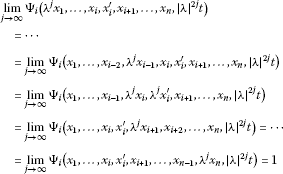(3.1)

and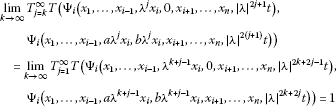(3.2)

for all ${x}_{1},\dots ,{x}_{i},{x}_{i}^{\prime },{x}_{i+1},\dots ,{x}_{n}\in \mathcal{X}$ and $t>0$. If $f:{\mathcal{X}}^{n}\to \mathcal{Y}$ is a mapping satisfying

$f\left({x}_{1},\dots ,{x}_{i-1},0,{x}_{i+1},\dots ,{x}_{n}\right)=0,$
(3.3)

and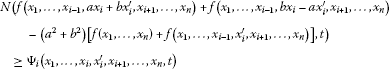(3.4)

for all ${x}_{1},\dots ,{x}_{i},{x}_{i}^{\prime },{x}_{i+1},\dots ,{x}_{n}\in \mathcal{X}$, $i\in \left\{1,2,\dots ,n\right\}$ and $t\in \left[0,\mathrm{\infty }\right)$, then for every $i\in \left\{1,2,\dots ,n\right\}$ there exists a unique general multi-Euler-Lagrange quadratic mapping ${Q}_{i}:{\mathcal{X}}^{n}\to \mathcal{Y}$ such that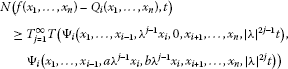(3.5)

for all ${x}_{1},\dots ,{x}_{n}\in \mathcal{X}$ and $t>0$.

Proof Fix ${x}_{1},\dots ,{x}_{n}\in \mathcal{X}$, $j\in \mathbb{N}\cup \left\{0\right\}$, $i\in \left\{1,2,\dots ,n\right\}$ and $t>0$. Putting ${x}_{i}^{\prime }=0$ in (3.4), we get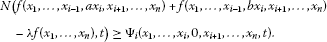(3.6)

Replacing ${x}_{i}$ by $a{x}_{i}$ and ${x}_{i}^{\prime }$ by $b{x}_{i}$ in (3.4), respectively, we have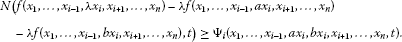(3.7)

From (3.6) and (3.7), one gets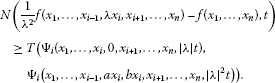(3.8)

Therefore one can get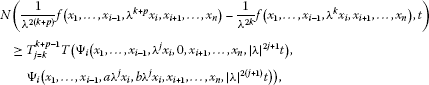and thus from (3.2) it follows that ${\left\{\frac{1}{{\lambda }^{2j}}f\left({x}_{1},\dots ,{x}_{i-1},{\lambda }^{j}{x}_{i},{x}_{i+1},\dots ,{x}_{n}\right)\right\}}_{j\in \mathbb{N}}$ is a Cauchy sequence in a complete non-Archimedean fuzzy normed space. Hence, we can define a mapping ${Q}_{i}:{\mathcal{X}}^{n}\to \mathcal{Y}$ such that

$\underset{j\to \mathrm{\infty }}{lim}N\left(\frac{1}{{\lambda }^{2j}}f\left({x}_{1},\dots ,{x}_{i-1},{\lambda }^{j}{x}_{i},{x}_{i+1},\dots ,{x}_{n}\right)-{Q}_{i}\left({x}_{1},\dots ,{x}_{n}\right),t\right)=1.$

Next, for each $k\in \mathbb{N}$ with $k\ge 1$, we have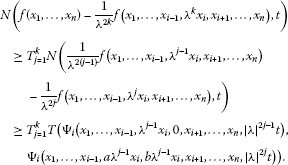Therefore,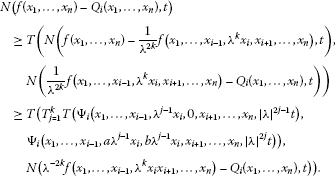Letting $k\to \mathrm{\infty }$ in this inequality, we obtain (3.5). Now, fix also ${x}_{i}^{\prime }\in \mathcal{X}$, from (3.1) and (3.4) it follows that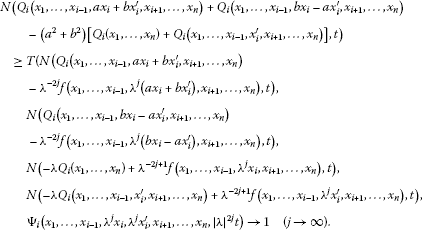Next, fix $k\in \left\{1,\dots ,n\right\}\mathrm{\setminus }\left\{i\right\}$, ${x}_{k}^{\prime }\in \mathcal{X}$, and assume, without loss of generality, that $k (the same arguments apply to the case where $k>i$). From (3.1) and (3.4), it follows that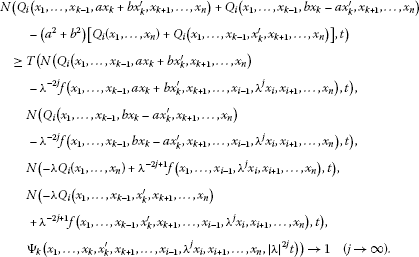Hence the mapping ${Q}_{i}$ is a general multi-Euler-Lagrange quadratic mapping. Let us finally assume that ${Q}_{i}^{\prime }:{\mathcal{X}}^{n}\to \mathcal{Y}$ is another multi-Euler-Lagrange quadratic mapping satisfying (3.5). Then, by (1.4), (3.5) and (3.2), it follows that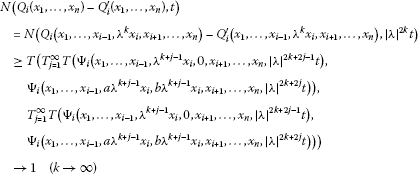and therefore ${Q}_{i}={Q}_{i}^{\prime }$. □

For $a=b$, we get the following result.

Theorem 3.2 Let $\mathbb{K}$ be a valued field, $\mathcal{X}$ be a vector space over $\mathbb{K}$ and $\left(\mathcal{Y},N,T\right)$ be a complete non-Archimedean fuzzy normed space over $\mathbb{K}$. Assume also that, for every $i\in \left\{1,2,\dots ,n\right\}$, ${\mathrm{\Psi }}_{i}:{\mathcal{X}}^{n+1}×\left[0,\mathrm{\infty }\right)\to \left[0,1\right]$ is a mapping such that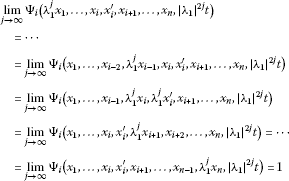(3.9)

and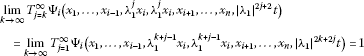(3.10)

for all ${x}_{1},\dots ,{x}_{i},{x}_{i}^{\prime },{x}_{i+1},\dots ,{x}_{n}\in \mathcal{X}$ and $t>0$. If $f:{\mathcal{X}}^{n}\to \mathcal{Y}$ is a mapping satisfying (3.3) and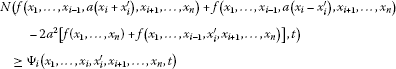(3.11)

for all ${x}_{1},\dots ,{x}_{i},{x}_{i}^{\prime },{x}_{i+1},\dots ,{x}_{n}\in \mathcal{X}$, $i\in \left\{1,2,\dots ,n\right\}$ and $t\in \left[0,\mathrm{\infty }\right)$, then for every $i\in \left\{1,2,\dots ,n\right\}$ there exists a unique general multi-Euler-Lagrange quadratic mapping ${Q}_{i}:{\mathcal{X}}^{n}\to \mathcal{Y}$ satisfying the functional equation (1.5) and such that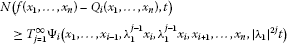(3.12)

for all ${x}_{1},\dots ,{x}_{n}\in \mathcal{X}$ and $t>0$.

Proof Fix ${x}_{1},\dots ,{x}_{n}\in \mathcal{X}$, $j\in \mathbb{N}\cup \left\{0\right\}$, $i\in \left\{1,2,\dots ,n\right\}$ and $t>0$. Putting ${x}_{i}^{\prime }={x}_{i}$ in (3.11), we get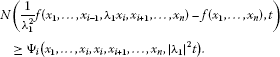(3.13)

Hence,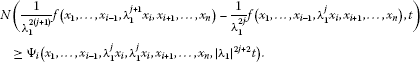Therefore one can get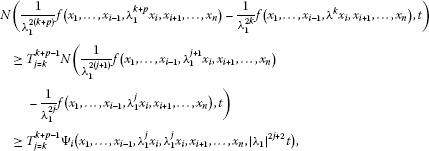and thus by (3.10) it follows that ${\left\{\frac{1}{{\lambda }_{1}^{2j}}f\left({x}_{1},\dots ,{x}_{i-1},{\lambda }_{1}^{j}{x}_{i},{x}_{i+1},\dots ,{x}_{n}\right)\right\}}_{j\in \mathbb{N}}$ is a Cauchy sequence in a complete non-Archimedean fuzzy normed space. Hence, we can define a mapping ${Q}_{i}:{\mathcal{X}}^{n}\to \mathcal{Y}$ such that

$\underset{j\to \mathrm{\infty }}{lim}N\left(\frac{1}{{\lambda }_{1}^{2j}}f\left({x}_{1},\dots ,{x}_{i-1},{\lambda }_{1}^{j}{x}_{i},{x}_{i+1},\dots ,{x}_{n}\right)-{Q}_{i}\left({x}_{1},\dots ,{x}_{n}\right),t\right)=1.$

Using (3.13) and induction, one can show that for any $k\in \mathbb{N}$ we have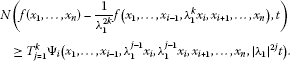Therefore,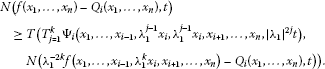Letting $k\to \mathrm{\infty }$ in this inequality, we obtain (3.12). The rest of the proof of this theorem is omitted as being similar to the corresponding that of Theorem 3.1. □

Let $\left(\mathcal{Y},N,T\right)$ be a complete non-Archimedean fuzzy normed space over a non-Archimedean field $\mathbb{K}$. In any such space, a sequence ${\left\{{x}_{k}\right\}}_{k\in \mathbb{N}}$ is Cauchy if and only if ${\left\{{x}_{k+1}-{x}_{k}\right\}}_{k\in \mathbb{N}}$ converges to zero. Analysis similar to that in the proof of Theorem 3.2 gives the following.

Theorem 3.3 Let $\mathbb{K}$ be a non-Archimedean field, $\mathcal{X}$ be a vector space over $\mathbb{K}$ and $\left(\mathcal{Y},N,T\right)$ be a complete non-Archimedean fuzzy normed space over $\mathbb{K}$. Assume also that, for every $i\in \left\{1,2,\dots ,n\right\}$, ${\mathrm{\Psi }}_{i}:{\mathcal{X}}^{n+1}×\left[0,\mathrm{\infty }\right)\to \left[0,1\right]$ is a mapping such that (3.9) holds and

$\underset{k\to \mathrm{\infty }}{lim}{T}_{j=1}^{\mathrm{\infty }}{\mathrm{\Psi }}_{i}\left({x}_{1},\dots ,{x}_{i-1},{\lambda }_{1}^{k+j-1}{x}_{i},{\lambda }_{1}^{k+j-1}{x}_{i},{x}_{i+1},\dots ,{x}_{n},{|{\lambda }_{1}|}^{2k+2j}t\right)=1$

for all ${x}_{1},\dots ,{x}_{i},{x}_{i}^{\prime },{x}_{i+1},\dots ,{x}_{n}\in \mathcal{X}$ and $t>0$. If $f:{\mathcal{X}}^{n}\to \mathcal{Y}$ is a mapping satisfying (3.3) and (3.11), then for every $i\in \left\{1,2,\dots ,n\right\}$ there exists a unique general multi-Euler-Lagrange quadratic mapping ${Q}_{i}:{\mathcal{X}}^{n}\to \mathcal{Y}$ satisfying (1.5) and (3.12).

Remark 3.4 Let $a,b\in \mathbb{N}$ and $\mathcal{X}$ be a commutative group, Theorems 3.1-3.3 also hold. For $a=1$, consider the non-Archimedean fuzzy normed space $\left(\mathcal{Y},{N}_{1},{T}_{M}\right)$ defined as in Example 2.6, Theorem 3.3 yields Theorem 2 in . If $a=b=±\frac{1}{\sqrt{2}}\in \mathbb{K}$, then ${\lambda }_{1}=2a=±\sqrt{2}\ne 1$ in Theorems 3.2-3.3 and $\lambda ={a}^{2}+{b}^{2}=1$ which is a singular case $\lambda =1$ of Theorem 3.1.

Analysis similar to that in the proof of Theorem 3.1 gives the following.

Theorem 3.5 Let $\mathbb{K}$ be a valued field, $\mathcal{X}$ be a vector space over $\mathbb{K}$ and $\left(\mathcal{Y},N,T\right)$ be a complete non-Archimedean fuzzy normed space over $\mathbb{K}$. Assume also that, for every $i\in \left\{1,2,\dots ,n\right\}$, ${\mathrm{\Psi }}_{i}:{\mathcal{X}}^{n+1}×\left[0,\mathrm{\infty }\right)\to \left[0,1\right]$ is a mapping such that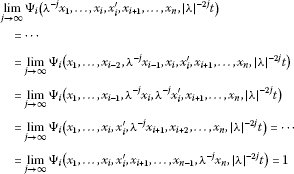(3.14)

and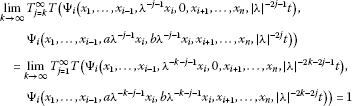(3.15)

for all ${x}_{1},\dots ,{x}_{i},{x}_{i}^{\prime },{x}_{i+1},\dots ,{x}_{n}\in \mathcal{X}$ and $t>0$. If $f:{\mathcal{X}}^{n}\to \mathcal{Y}$ is a mapping satisfying (3.3) and (3.4), then for every $i\in \left\{1,2,\dots ,n\right\}$ there exists a unique general multi-Euler-Lagrange quadratic mapping ${Q}_{i}:{\mathcal{X}}^{n}\to \mathcal{Y}$ such that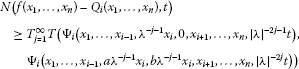for all ${x}_{1},\dots ,{x}_{n}\in \mathcal{X}$ and $t>0$.

Corollary 3.6 Let $\mathbb{K}$ be a non-Archimedean field with $0<|\lambda |<1$, $\mathcal{X}$ be a normed space over $\mathbb{K}$ and let $\left(\mathcal{Y},N,T\right)$ be a complete non-Archimedean fuzzy normed space over $\mathbb{K}$ under a t-norm $T\in \mathcal{H}$. Assume also that $\delta >0$ and $\alpha :\left[0,\mathrm{\infty }\right)\to \left[0,\mathrm{\infty }\right)$ is a function such that $\alpha \left({|\lambda |}^{-1}\right)<{|\lambda |}^{-1}$ and $\alpha \left({|\lambda |}^{-1}t\right)\le \alpha \left({|\lambda |}^{-1}\right)\alpha \left(t\right)$ for all $t\in \left[0,\mathrm{\infty }\right)$.

If $f:{\mathcal{X}}^{n}\to \mathcal{Y}$ is a mapping satisfying (3.3) and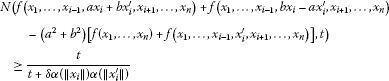(3.16)

for all ${x}_{1},\dots ,{x}_{i},{x}_{i}^{\prime },{x}_{i+1},\dots ,{x}_{n}\in \mathcal{X}$, $i\in \left\{1,2,\dots ,n\right\}$ and $t\in \left[0,\mathrm{\infty }\right)$, then for every $i\in \left\{1,2,\dots ,n\right\}$ there exists a unique general multi-Euler-Lagrange quadratic mapping ${Q}_{i}:{\mathcal{X}}^{n}\to \mathcal{Y}$ such that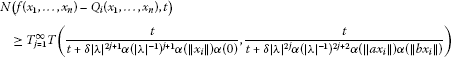for all ${x}_{1},\dots ,{x}_{n}\in \mathcal{X}$ and $t>0$.

Proof Fix $i\in \left\{1,2,\dots ,n\right\}$, ${x}_{1},\dots ,{x}_{i},{x}_{i}^{\prime },{x}_{i+1},\dots ,{x}_{n}\in \mathcal{X}$ and $t\in \left[0,\mathrm{\infty }\right)$. Let ${\mathrm{\Psi }}_{i}:{\mathcal{X}}^{n+1}×\left[0,\mathrm{\infty }\right)\to \left[0,1\right]$ be defined by ${\mathrm{\Psi }}_{i}\left({x}_{1},\dots ,{x}_{i},{x}_{i}^{\prime },{x}_{i+1},\dots ,{x}_{n},t\right):=\frac{t}{t+\delta \alpha \left(\parallel {x}_{i}\parallel \right)\alpha \left(\parallel {x}_{i}^{\prime }\parallel \right)}$. Then we can apply Theorem 3.5 to obtain the result. □

Remark 3.7 Let $0<|\lambda |<1$ and $p\in \left(0,1\right)$. Then the mapping $\alpha :\left[0,\mathrm{\infty }\right)\to \left[0,\mathrm{\infty }\right)$ given by $\alpha \left(t\right):={t}^{p}$, $t\in \left[0,\mathrm{\infty }\right)$ satisfies $\alpha \left({|\lambda |}^{-1}\right)<{|\lambda |}^{-1}$ and $\alpha \left({|\lambda |}^{-1}t\right)\le \alpha \left({|\lambda |}^{-1}\right)\alpha \left(t\right)$ for all $t\in \left[0,\mathrm{\infty }\right)$.

## 4 Stability of the functional equation (1.1): a fixed point method

Throughout this section, we prove the stability of Eq. (1.1) in complete non-Archimedean fuzzy normed spaces using the fixed point method.

Theorem 4.1 Let $\mathbb{K}$ be a valued field, $\mathcal{X}$ be a vector space over $\mathbb{K}$ and $\left(\mathcal{Y},N,T\right)$ be a complete non-Archimedean fuzzy normed space over $\mathbb{K}$. Assume also that, for every $i\in \left\{1,2,\dots ,n\right\}$, ${\mathrm{\Psi }}_{i}:{\mathcal{X}}^{n+1}×\left[0,\mathrm{\infty }\right)\to \left[0,1\right]$ is a mapping such that (3.1) holds and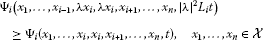(4.1)

for an ${L}_{i}\in \left(0,1\right)$. If $f:{\mathcal{X}}^{n}\to \mathcal{Y}$ is a mapping satisfying (3.3) and (3.4), then for every $i\in \left\{1,2,\dots ,n\right\}$ there exists a unique general multi-Euler-Lagrange quadratic mapping ${Q}_{i}:{\mathcal{X}}^{n}\to \mathcal{Y}$ such that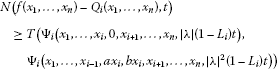(4.2)

for all ${x}_{1},\dots ,{x}_{n}\in \mathcal{X}$ and $t>0$.

Proof Fix an $i\in \left\{1,2,\dots ,n\right\}$. Consider the set $\mathrm{\Omega }:=\left\{g:{\mathcal{X}}^{n}\to \mathcal{Y}\right\}$ and introduce the generalized metric on Ω:

$\begin{array}{rcl}{d}_{i}\left(g,h\right)& =& inf\left\{C\in \left[0,\mathrm{\infty }\right]:N\left(g\left({x}_{1},\dots ,{x}_{n}\right)-h\left({x}_{1},\dots ,{x}_{n}\right),Ct\right)\\ \ge T\left({\mathrm{\Psi }}_{i}\left({x}_{1},\dots ,{x}_{i},0,{x}_{i+1},\dots ,{x}_{n},|\lambda |t\right),\\ {\mathrm{\Psi }}_{i}\left({x}_{1},\dots ,{x}_{i-1},a{x}_{i},b{x}_{i},{x}_{i+1},\dots ,{x}_{n},{|\lambda |}^{2}t\right)\right),\\ {x}_{1},\dots ,{x}_{n}\in \mathcal{X},t>0\right\},\phantom{\rule{1em}{0ex}}g,h\in \mathrm{\Omega }.\end{array}$

A standard verification (see for instance ) shows that $\left(\mathrm{\Omega },{d}_{i}\right)$ is a complete generalized metric space. We now define an operator ${J}_{i}:\mathrm{\Omega }\to \mathrm{\Omega }$ by

${J}_{i}g\left({x}_{1},\dots ,{x}_{n}\right)=\frac{1}{{\lambda }^{2}}g\left({x}_{1},\dots ,{x}_{i-1},\lambda {x}_{i},{x}_{i+1},\dots ,{x}_{n}\right),\phantom{\rule{1em}{0ex}}g\in \mathrm{\Omega },{x}_{1},\dots ,{x}_{n}\in \mathcal{X}.$

Let $g,h\in \mathrm{\Omega }$ and ${C}_{g,h}\in \left[0,\mathrm{\infty }\right]$ with ${d}_{i}\left(g,h\right)\le {C}_{g,h}$. Then

$\begin{array}{rcl}N\left(g\left({x}_{1},\dots ,{x}_{n}\right)-h\left({x}_{1},\dots ,{x}_{n}\right),{C}_{g,h}t\right)& \ge & T\left({\mathrm{\Psi }}_{i}\left({x}_{1},\dots ,{x}_{i},0,{x}_{i+1},\dots ,{x}_{n},|\lambda |t\right),\\ {\mathrm{\Psi }}_{i}\left({x}_{1},\dots ,{x}_{i-1},a{x}_{i},b{x}_{i},{x}_{i+1},\dots ,{x}_{n},{|\lambda |}^{2}t\right)\right),\end{array}$

which together with (4.1) gives

$\begin{array}{rcl}N\left({J}_{i}g\left({x}_{1},\dots ,{x}_{n}\right)-{J}_{i}h\left({x}_{1},\dots ,{x}_{n}\right),t\right)& \ge & T\left({\mathrm{\Psi }}_{i}\left({x}_{1},\dots ,{x}_{i},0,{x}_{i+1},\dots ,{x}_{n},\frac{|\lambda |t}{{L}_{i}{C}_{g,h}}\right),\\ {\mathrm{\Psi }}_{i}\left({x}_{1},\dots ,{x}_{i-1},a{x}_{i},b{x}_{i},{x}_{i+1},\dots ,{x}_{n},\frac{{|\lambda |}^{2}t}{{L}_{i}{C}_{g,h}}\right)\right),\end{array}$

and consequently, ${d}_{i}\left({J}_{i}g,{J}_{i}h\right)\le {L}_{i}{C}_{g,h}$, which means that the operator ${J}_{i}$ is strictly contractive. Moreover, from (3.8) it follows that

$\begin{array}{rcl}N\left({J}_{i}f\left({x}_{1},\dots ,{x}_{n}\right)-f\left({x}_{1},\dots ,{x}_{n}\right),t\right)& \ge & T\left({\mathrm{\Psi }}_{i}\left({x}_{1},\dots ,{x}_{i},0,{x}_{i+1},\dots ,{x}_{n},|\lambda |t\right),\\ {\mathrm{\Psi }}_{i}\left({x}_{1},\dots ,{x}_{i-1},a{x}_{i},b{x}_{i},{x}_{i+1},\dots ,{x}_{n},{|\lambda |}^{2}t\right)\right)\end{array}$

and thus ${d}_{i}\left({J}_{i}f,f\right)\le 1<\mathrm{\infty }$. Therefore, by Theorem 2.8, ${J}_{i}$ has a unique fixed point ${Q}_{i}:{\mathcal{X}}^{n}\to \mathcal{Y}$ in the set ${\mathrm{\Omega }}^{\ast }=\left\{g\in \mathrm{\Omega }:d\left(f,g\right)<\mathrm{\infty }\right\}$ such that

$\frac{1}{{\lambda }^{2}}{Q}_{i}\left({x}_{1},\dots ,{x}_{i-1},\lambda {x}_{i},{x}_{i+1},\dots ,{x}_{n}\right)={Q}_{i}\left({x}_{1},\dots ,{x}_{n}\right)$
(4.3)

and

${Q}_{i}\left({x}_{1},\dots ,{x}_{n}\right)=\underset{j\to \mathrm{\infty }}{lim}\frac{1}{{\lambda }^{2j}}f\left({x}_{1},\dots ,{x}_{i-1},{\lambda }^{j}{x}_{i},{x}_{i+1},\dots ,{x}_{n}\right).$

Furthermore, from the fact that $f\in {\mathrm{\Omega }}^{\ast }$, Theorem 2.8, and ${d}_{i}\left({J}_{i}f,f\right)\le 1$, we get

${d}_{i}\left(f,{Q}_{i}\right)\le \frac{1}{1-{L}_{i}}{d}_{i}\left({J}_{i}f,f\right)\le \frac{1}{1-{L}_{i}}$

and (4.2) follows. Similar to the proof of Theorem 3.1, one can prove that the mapping ${Q}_{i}$ is also general multi-Euler-Lagrange quadratic.

Let us finally assume that ${Q}_{i}^{\prime }:{\mathcal{X}}^{n}\to \mathcal{Y}$ is a general multi-Euler-Lagrange quadratic mapping satisfying condition (4.2). Then ${Q}_{i}^{\prime }$ fulfills (4.3), and therefore, it is a fixed point of the operator ${J}_{i}$. Moreover, by (4.2), we have ${d}_{i}\left(f,{Q}_{i}^{\prime }\right)\le \frac{1}{1-{L}_{i}}<\mathrm{\infty }$, and consequently ${Q}_{i}^{\prime }\in {\mathrm{\Omega }}^{\ast }$. Theorem 2.8 shows that ${Q}_{i}^{\prime }={Q}_{i}$. □

Similar to Theorem 4.1, one can prove the following result.

Theorem 4.2 Let $\mathbb{K}$ be a valued field, $\mathcal{X}$ be a vector space over $\mathbb{K}$ and $\left(\mathcal{Y},N,T\right)$ be a complete non-Archimedean fuzzy normed space over $\mathbb{K}$. Assume also that, for every $i\in \left\{1,2,\dots ,n\right\}$, ${\mathrm{\Psi }}_{i}:{\mathcal{X}}^{n+1}×\left[0,\mathrm{\infty }\right)\to \left[0,1\right]$ is a mapping such that (3.14) holds and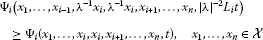for an ${L}_{i}\in \left(0,1\right)$. If $f:{\mathcal{X}}^{n}\to \mathcal{Y}$ is a mapping satisfying (3.3) and (3.4), then for every $i\in \left\{1,2,\dots ,n\right\}$ there exists a unique general multi-Euler-Lagrange quadratic mapping ${Q}_{i}:{\mathcal{X}}^{n}\to \mathcal{Y}$ such that

$\begin{array}{rcl}N\left(f\left({x}_{1},\dots ,{x}_{n}\right)-{Q}_{i}\left({x}_{1},\dots ,{x}_{n}\right),t\right)& \ge & T\left({\mathrm{\Psi }}_{i}\left({x}_{1},\dots ,{x}_{i},0,{x}_{i+1},\dots ,{x}_{n},|\lambda |\left({L}_{i}^{-1}-1\right)t\right),\\ {\mathrm{\Psi }}_{i}\left({x}_{1},\dots ,{x}_{i-1},a{x}_{i},b{x}_{i},{x}_{i+1},\dots ,{x}_{n},{|\lambda |}^{2}\left({L}_{i}^{-1}-1\right)t\right)\right)\end{array}$

for all ${x}_{1},\dots ,{x}_{n}\in \mathcal{X}$ and $t>0$.

Remark 4.3 Similar to the proof of Corollary 3.6, one can deduce from Theorem 4.2 an analog of Corollary 3.6.

As applications of Theorems 4.1 and 4.2 , we get the following corollaries.

Corollary 4.4 Let $\mathcal{X}$ be a real normed space, $\mathcal{Y}$ be a real Banach space and $\left(\mathcal{Y},N,{T}_{P}\right)$ be the complete non-Archimedean fuzzy normed space defined as in the second example in the preliminaries. Let $\delta ,r,p,q\in \left(0,\mathrm{\infty }\right)$ such that $r,s:=p+q\in \left(2,\mathrm{\infty }\right)$, or $r,s\in \left(0,2\right)$. If $f:{\mathcal{X}}^{n}\to \mathcal{Y}$ is a mapping satisfying (3.3) and (3.4), where

${\mathrm{\Psi }}_{i}\left({x}_{1},\dots ,{x}_{i},{x}_{i}^{\prime },{x}_{i+1},\dots ,{x}_{n},t\right):=\frac{t}{t+\delta \left[{\parallel {x}_{1}\parallel }^{r}\cdots {\parallel {x}_{i-1}\parallel }^{r}\left({\parallel {x}_{i}\parallel }^{p}{\parallel {x}_{i}^{\prime }\parallel }^{q}\right){\parallel {x}_{i+1}\parallel }^{r}\cdots {\parallel {x}_{n}\parallel }^{r}\right]},$

then for every $i\in \left\{1,2,\dots ,n\right\}$ there exists a unique general multi-Euler-Lagrange quadratic mapping ${Q}_{i}:{\mathcal{X}}^{n}\to \mathcal{Y}$ such that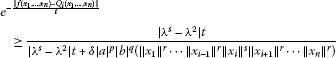(4.4)

for all ${x}_{1},\dots ,{x}_{n}\in \mathcal{X}$ and $t>0$.

Proof Fix $i\in \left\{1,2,\dots ,n\right\}$, ${x}_{1},\dots ,{x}_{i},{x}_{i}^{\prime },{x}_{i+1},\dots ,{x}_{n}\in \mathcal{X}$, $t\in \left[0,\mathrm{\infty }\right)$ and assume that $\lambda >1$, $r,s\in \left(2,\mathrm{\infty }\right)$ (the same arguments apply to the case where $\lambda <1$, $r,s\in \left(0,2\right)$). Then we can choose ${L}_{i}={\lambda }^{2-s}<1$ and apply Theorem 4.2 to obtain the result. For $\lambda >1$, $r,s\in \left(0,2\right)$, or $\lambda <1$, $r,s\in \left(2,\mathrm{\infty }\right)$, the corollary follows from Theorem 4.1. □

Corollary 4.5 Let $\mathcal{X}$ be a real normed space and $\mathcal{Y}$ be a real Banach space (or $\mathcal{X}$ be a non-Archimedean normed space and $\mathcal{Y}$ be a complete non-Archimedean normed space over a non-Archimedean field $\mathbb{K}$, respectively). Let $\delta >0$ and $r\in \left(0,2\right)\cup \left(2,\mathrm{\infty }\right)$. If $f:{\mathcal{X}}^{n}\to \mathcal{Y}$ is a mapping satisfying (3.3) and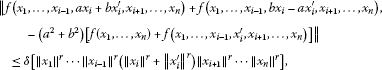then for every $i\in \left\{1,2,\dots ,n\right\}$ there exists a unique general multi-Euler-Lagrange quadratic mapping ${Q}_{i}:{\mathcal{X}}^{n}\to \mathcal{Y}$ such that

$\parallel f\left({x}_{1},\dots ,{x}_{n}\right)-{Q}_{i}\left({x}_{1},\dots ,{x}_{n}\right)\parallel \le \frac{max\left\{|\lambda |,{|a|}^{r}+{|b|}^{r}\right\}\delta \left({\parallel {x}_{1}\parallel }^{r}\cdots {\parallel {x}_{n}\parallel }^{r}\right)}{|{|\lambda |}^{r}-{|\lambda |}^{2}|}$

for all ${x}_{1},\dots ,{x}_{n}\in \mathcal{X}$.

Proof Consider the non-Archimedean fuzzy normed space $\left(\mathcal{Y},{N}_{1},{T}_{M}\right)$ defined as in the first example in the preliminaries, ${\mathrm{\Psi }}_{i}$ be defined by

${\mathrm{\Psi }}_{i}\left({x}_{1},\dots ,{x}_{i},{x}_{i}^{\prime },{x}_{i+1},\dots ,{x}_{n},t\right):=\frac{t}{t+\delta \left[{\parallel {x}_{1}\parallel }^{r}\cdots {\parallel {x}_{i-1}\parallel }^{r}\left({\parallel {x}_{i}\parallel }^{r}+{\parallel {x}_{i}^{\prime }\parallel }^{r}\right){\parallel {x}_{i+1}\parallel }^{r}\cdots {\parallel {x}_{n}\parallel }^{r}\right]},$

and apply Theorems 4.1 and 4.2.

The following example shows that the Hyers-Ulam stability problem for the case of $r=2$ was excluded in Corollary 4.5. □

Example 4.6 Let $\varphi :\mathbb{C}\to \mathbb{C}$ be defined by

Consider the function $f:\mathbb{C}\to \mathbb{C}$ be defined by

$f\left(x\right)=\sum _{j=0}^{\mathrm{\infty }}\frac{\varphi \left({\alpha }^{j}x\right)}{{\alpha }^{2j}}$

for all $x\in \mathbb{C}$, where $\alpha >max\left\{|a|,|b|,1\right\}$. Then f satisfies the functional inequality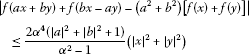(4.5)

for all $x,y\in \mathbb{C}$, but there do not exist a general multi-Euler-Lagrange quadratic function $Q:\mathbb{C}\to \mathbb{C}$ and a constant $d>0$ such that $|f\left(x\right)-Q\left(x\right)|\le d{|x|}^{2}$ for all $x\in \mathbb{C}$.

It is clear that f is bounded by $\frac{{\alpha }^{2}}{{\alpha }^{2}-1}$ on $\mathbb{C}$. If ${|x|}^{2}+{|y|}^{2}=0$ or ${|x|}^{2}+{|y|}^{2}\ge \frac{1}{{\alpha }^{2}}$, then

$|f\left(ax+by\right)+f\left(bx-ay\right)-\left({a}^{2}+{b}^{2}\right)\left[f\left(x\right)+f\left(y\right)\right]|\le \frac{2{\alpha }^{4}\left({|a|}^{2}+{|b|}^{2}+1\right)}{{\alpha }^{2}-1}\left({|x|}^{2}+{|y|}^{2}\right).$

Now suppose that $0<{|x|}^{2}+{|y|}^{2}<\frac{1}{{\alpha }^{2}}$. Then there exists an integer $k\ge 1$ such that

$\frac{1}{{\alpha }^{2\left(k+2\right)}}\le {|x|}^{2}+{|y|}^{2}<\frac{1}{{\alpha }^{2\left(k+1\right)}}.$
(4.6)

Hence

${\alpha }^{l}|ax+by|<1,\phantom{\rule{2em}{0ex}}{\alpha }^{l}|bx-ay|<1,\phantom{\rule{2em}{0ex}}{\alpha }^{l}|x|<1,\phantom{\rule{2em}{0ex}}{\alpha }^{l}|y|<1$

for all $l=0,1,\dots ,k-1$. From the definition of f and the inequality (4.6), we obtain that f satisfies (4.5). Now, we claim that the functional equation (1.1) is not stable for $r=2$ in Corollary 4.5. Suppose, on the contrary, that there exist a general multi-Euler-Lagrange quadratic function $Q:\mathbb{C}\to \mathbb{C}$ and a constant $d>0$ such that $|f\left(x\right)-Q\left(x\right)|\le d{|x|}^{2}$ for all $x\in \mathbb{C}$. Then there exists a constant $c\in \mathbb{C}$ such that $Q\left(x\right)=c{x}^{2}$ for all rational numbers x. So, we obtain that

$|f\left(x\right)|\le \left(d+|c|\right){|x|}^{2}$
(4.7)

for all rational numbers x. Let $s\in \mathbb{N}$ with $s+1>d+|c|$. If x is a rational number in $\left(0,{\alpha }^{-s}\right)$, then ${\alpha }^{j}x\in \left(0,1\right)$ for all $j=0,1,\dots ,s$, and for this x we get

$f\left(x\right)=\sum _{j=0}^{\mathrm{\infty }}\frac{\varphi \left({\alpha }^{j}x\right)}{{\alpha }^{2j}}\ge \sum _{j=0}^{s}\frac{\varphi \left({\alpha }^{j}x\right)}{{\alpha }^{2j}}=\left(s+1\right){x}^{2}>\left(d+|c|\right){x}^{2},$

Corollary 4.7 Let $\mathbb{K}$ be a non-Archimedean field with $0<|\lambda |<1$, $\mathcal{X}$ be a normed space over $\mathbb{K}$ and $\mathcal{Y}$ be a complete non-Archimedean normed space over $\mathbb{K}$. Let $\delta ,p,q\in \left(0,\mathrm{\infty }\right)$ such that $p+q\in \left(0,2\right)$. If $f:{\mathcal{X}}^{n}\to \mathcal{Y}$ is a mapping satisfying (3.3) and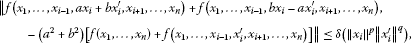then for every $i\in \left\{1,2,\dots ,n\right\}$ there exists a unique general multi-Euler-Lagrange quadratic mapping ${Q}_{i}:{\mathcal{X}}^{n}\to \mathcal{Y}$ such that

$\parallel f\left({x}_{1},\dots ,{x}_{n}\right)-{Q}_{i}\left({x}_{1},\dots ,{x}_{n}\right)\parallel \le \frac{\delta {|a|}^{p}{|b|}^{q}{\parallel {x}_{i}\parallel }^{p+q}}{{|\lambda |}^{p+q}-{|\lambda |}^{2}}$
(4.8)

for all ${x}_{1},\dots ,{x}_{n}\in \mathcal{X}$.

Proof Fix $i\in \left\{1,2,\dots ,n\right\}$, ${x}_{1},\dots ,{x}_{i},{x}_{i}^{\prime },{x}_{i+1},\dots ,{x}_{n}\in \mathcal{X}$ and $t\in \left[0,\mathrm{\infty }\right)$. Let ${\mathrm{\Psi }}_{i}:{\mathcal{X}}^{n+1}×\left[0,\mathrm{\infty }\right)\to \left[0,1\right]$ be defined by ${\mathrm{\Psi }}_{i}\left({x}_{1},\dots ,{x}_{i},{x}_{i}^{\prime },{x}_{i+1},\dots ,{x}_{n},t\right):=\frac{t}{t+\delta \left({\parallel {x}_{i}\parallel }^{p}{\parallel {x}_{i}^{\prime }\parallel }^{q}\right)}$. Consider the non-Archimedean fuzzy normed space $\left(\mathcal{Y},{N}_{1},{T}_{M}\right)$ defined as in Example 2.6, and apply Theorem 4.2. □

Corollary 4.8 Let $\mathcal{X}$ be a real normed space and $\mathcal{Y}$ be a real Banach space. Let $\delta ,r,p,q\in \left(0,\mathrm{\infty }\right)$ such that $r,p+q\in \left(0,2\right)$, or $r,p+q\in \left(2,\mathrm{\infty }\right)$. If $f:{\mathcal{X}}^{n}\to \mathcal{Y}$ is a mapping satisfying (3.3) and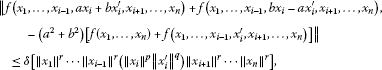then, for every $i\in \left\{1,2,\dots ,n\right\}$, there exists a unique general multi-Euler-Lagrange quadratic mapping ${Q}_{i}:{\mathcal{X}}^{n}\to \mathcal{Y}$ such that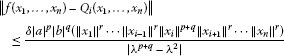(4.9)

for all ${x}_{1},\dots ,{x}_{n}\in \mathcal{X}$.

Proof Fix $i\in \left\{1,2,\dots ,n\right\}$, ${x}_{1},\dots ,{x}_{i},{x}_{i}^{\prime },{x}_{i+1},\dots ,{x}_{n}\in \mathcal{X}$ and $t\in \left[0,\mathrm{\infty }\right)$. Let ${\mathrm{\Psi }}_{i}:{\mathcal{X}}^{n+1}×\left[0,\mathrm{\infty }\right)\to \left[0,1\right]$ be defined by

${\mathrm{\Psi }}_{i}\left({x}_{1},\dots ,{x}_{i},{x}_{i}^{\prime },{x}_{i+1},\dots ,{x}_{n},t\right):=\frac{t}{t+\delta \left[{\parallel {x}_{1}\parallel }^{r}\cdots {\parallel {x}_{i-1}\parallel }^{r}\left({\parallel {x}_{i}\parallel }^{p}{\parallel {x}_{i}^{\prime }\parallel }^{q}\right){\parallel {x}_{i+1}\parallel }^{r}\cdots {\parallel {x}_{n}\parallel }^{r}\right]}.$

Consider the non-Archimedean fuzzy normed space $\left(\mathcal{Y},{N}_{1},{T}_{M}\right)$ defined as in Example 2.6, and apply Theorems 4.1 and 4.2. □

Remark 4.9 Theorems 4.1 and 4.2 can be regarded as a generalization of the classical stability result in the framework of normed spaces (see ). For $a=b=1$ and $n=1$, Corollary 4.8 yields the main theorem in . The generalized Hyers-Ulam stability problem for the case of $r=p+q=2$ was excluded in Corollary 4.8 (see).

Note that by (4.4) one can getLetting $t\to \mathrm{\infty }$ in this inequality, we obtain (4.9). Thus Corollary 4.8 is a singular case of Corollary 4.4. This study indeed presents a relationship between three various disciplines: the theory of non-Archimedean fuzzy normed spaces, the theory of stability of functional equations and the fixed point theory.

## References

1. Agarwal RP, Xu B, Zhang W: Stability of functional equations in single variable. J. Math. Anal. Appl. 2003, 288: 852–869. 10.1016/j.jmaa.2003.09.032

2. Aoki T: On the stability of the linear transformation in Banach spaces. J. Math. Soc. Jpn. 1950, 2: 64–66. 10.2969/jmsj/00210064

3. Cho YJ, Grabiec M, Radu V: On Nonsymmetric Topological and Probabilistic Structures. Nova Science Publishers, New York; 2006.

4. Cho YJ, Park C, Saadati R: Functional inequalities in non-Archimedean Banach spaces. Appl. Math. Lett. 2010, 23: 1238–1242. 10.1016/j.aml.2010.06.005

5. Cho YJ, Saadati R: Lattictic non-Archimedean random stability of ACQ functional equation. Adv. Differ. Equ. 2011, 2011: 31. 10.1186/1687-1847-2011-31

6. Ebadian A, Ghobadipour N, Gordji ME: On the stability of a parametric additive functional equation in quasi-Banach spaces. Abstr. Appl. Anal. 2012., 2012: Article ID 235359

7. Ciepliński K: On the generalized Hyers-Ulam stability of multi-quadratic mappings. Comput. Math. Appl. 2011, 62: 3418–3426. 10.1016/j.camwa.2011.08.057

8. Diaz JB, Margolis B: A fixed point theorem of the alternative for the contractions on generalized complete metric space. Bull. Am. Math. Soc. 1968, 74: 305–309. 10.1090/S0002-9904-1968-11933-0

9. Găvruţa P: A generalization of the Hyers-Ulam-Rassias stability of approximately additive mappings. J. Math. Anal. Appl. 1994, 184: 431–436. 10.1006/jmaa.1994.1211

10. Găvruţa L, Găvruţa P: On a problem of John M. Rassias concerning the stability in Ulam sense of Euler-Lagrange equation. In Functional Equations, Difference Inequalities and Ulam Stability Notions (F.U.N.). Edited by: JM Rassias. Nova Science Publishers, New York; 2010:47–53. Chapter 4

11. Gordji ME, Ghaemi MB, Cho YJ, Majani H: A general system of Euler-Lagrange-type quadratic functional equations in Menger probabilistic non-Archimedean 2-normed spaces. Abstr. Appl. Anal. 2011., 2011: Article ID 208163

12. Hadz̆ić O, Pap E, Budincević M: Countable extension of triangular norms and their applications to the fixed point theory in probabilistic metric spaces. Kybernetika 2002, 38: 363–381.

13. Hyers DH: On the stability of the linear functional equation. Proc. Natl. Acad. Sci. USA 1941, 27: 222–224. 10.1073/pnas.27.4.222

14. Hyers DH, Isac G, Rassias TM: Stability of Functional Equations in Several Variables. Birkhäuser, Basel; 1998.

15. Kenary HA, Rezaei H, Talebzadeh S, Park C:Stability of the Jensen equation in ${C}^{\ast }$-algebras: a fixed point approach. Adv. Differ. Equ. 2012, 2012: 17. 10.1186/1687-1847-2012-17

16. Kenary HA, Lee J, Park C: Nonlinear approximation of an ACQ-functional equation in nan-spaces. Fixed Point Theory Appl. 2011, 2011: 60. 10.1186/1687-1812-2011-60

17. Rassias JM: On the stability of the Euler-Lagrange functional equation. Chin. J. Math. 1992, 20: 185–190.

18. Jung SM: Hyers-Ulam-Rassias Stability of Functional Equations in Nonlinear Analysis. Springer, New York; 2011.

19. Jung SM:A fixed point approach to the stability of the equation $f\left(x+y\right)=\frac{f\left(x\right)f\left(y\right)}{f\left(x\right)+f\left(y\right)}$. Aust. J. Math. Anal. Appl. 2009, 6: 8.

20. Kannappan Pl: Functional Equations and Inequalities with Applications. Springer, New York; 2009.

21. Khrennikov A: Non-Archimedean Analysis: Quantum Paradoxes, Dynamical Systems and Biological Models. Kluwer Academic, Dordrecht; 1997.

22. Miheţ D: The stability of the additive Cauchy functional equation in non-Archimedean fuzzy normed spaces. Fuzzy Sets Syst. 2010, 161: 2206–2212. 10.1016/j.fss.2010.02.010

23. Mirmostafaee AK, Moslehian MS: Stability of additive mappings in non-Archimedean fuzzy normed spaces. Fuzzy Sets Syst. 2009, 160: 1643–1652. 10.1016/j.fss.2008.10.011

24. Park C: Multi-quadratic mappings in Banach spaces. Proc. Am. Math. Soc. 2003, 131: 2501–2504. 10.1090/S0002-9939-02-06886-7

25. Park C: Fixed points and the stability of an AQCQ-functional equation in non-Archimedean normed spaces. Abstr. Appl. Anal. 2010., 2010: Article ID 849543

26. Radu V: The fixed point alternative and the stability of functional equations. Fixed Point Theory 2003, 4: 91–96.

27. Rassias TM: On the stability of the linear mapping in Banach spaces. Proc. Am. Math. Soc. 1978, 72: 297–300. 10.1090/S0002-9939-1978-0507327-1

28. Saadati R, Cho YJ, Vahidi J: The stability of the quartic functional equation in various spaces. Comput. Math. Appl. 2010, 60: 1994–2002. 10.1016/j.camwa.2010.07.034

29. Schweizer B, Sklar A: Probabilistic Metric Spaces. North-Holland, New York; 1983.

30. Ulam SM: A Collection of the Mathematical Problems. Interscience, New York; 1960.

31. Xu TZ, Rassias JM, Xu WX: Stability of a general mixed additive-cubic functional equation in non-Archimedean fuzzy normed spaces. J. Math. Phys. 2010., 51: Article ID 093508

32. Xu TZ: Stability of multi-Jensen mappings in non-Archimedean normed spaces. J. Math. Phys. 2012., 53: Article ID 023507

33. Xu TZ: On the stability of multi-Jensen mappings in β -normed spaces. Appl. Math. Lett. 2012, 25: 1866–1870. doi:10.1016/j.aml.2012.02.049 10.1016/j.aml.2012.02.049

34. Xu TZ, Rassias JM: On the Hyers-Ulam stability of a general mixed additive and cubic functional equation in n -Banach spaces. Abstr. Appl. Anal. 2012., 2012: Article ID 926390

35. Xu TZ, Rassias JM: A fixed point approach to the stability of an AQ-functional equation on β -Banach modules. Fixed Point Theory Appl. 2012., 2012: Article ID 32

36. Alotaibi A, Mohiuddine SA: On the stability of a cubic functional equation in random 2-normed spaces. Adv. Differ. Equ. 2012, 2012: 39. 10.1186/1687-1847-2012-39

37. Mohiuddine SA, Alotaibi A: Fuzzy stability of a cubic functional equation via fixed point technique. Adv. Differ. Equ. 2012, 2012: 48. 10.1186/1687-1847-2012-48

## Acknowledgements

The first author was supported by the National Natural Science Foundation of China (NNSFC) (Grant No. 11171022).

## Author information

Authors

### Corresponding author

Correspondence to Tian Zhou Xu.

### Competing interests

The authors declare that they have no competing interests.

### Authors’ contributions

All authors carried out the proof. All authors conceived of the study and participated in its design and coordination. All authors read and approved the final manuscript.

## Rights and permissions

Reprints and Permissions

Xu, T.Z., Rassias, J.M. Stability of general multi-Euler-Lagrange quadratic functional equations in non-Archimedean fuzzy normed spaces. Adv Differ Equ 2012, 119 (2012). https://doi.org/10.1186/1687-1847-2012-119